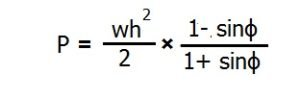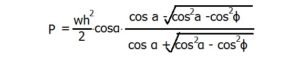# Retaining wall design

Refer :- http://www.dailycivil.com

## RETAINING WALL DESIGN:The thrust from the backing which tends to overturn the wall or causes it to slide is considered as the deciding factor in the selection of the section and type of the retaining wall.
The thrust by the backing depends on several conditions such as cohesion of soil, dryness of the backing material, the manner in which the material is filled against the wall etc.

Having known The thrust the section is so designed that the self-weight is enough to resist the thrust to slide the wall and the bottom width of the wall is such that the resultant force (resultant of the weight of wall and pressure of filling behind) lies within the middle third of the base.
This condition is essentially required to prevent the tendency of the thrust to overturn the wall and to ensure that there is no tension at the wall base. It is equally essential to ascertain that the maximum stress at the toe of the wall does not exceed the safe bearing capacity of the soil.

## CALCULATION OF EARTH PRESSURE:

The thrust due to the back filling, which may be assumed to be earth, is generally calculated by Rankine’s theory. The theory is based on the assumption that the backing material or earth consists of cohesionless granular particles. The formulae derived from this theory under different conditions of backfilling are given below:
Case 1: Walls with earth levelled with the top of the wall:
a) Horizontal pressure per sq.m (ph) at a depth of (h) meter below the levelled top is given by the following formula:Where
w = Weight of filling in kg/m3
ϕ = Angle of repose of the soil.
b) Total horizontal pressure (P) at a depth of (h) meter per meter length of the wall is given by the following formula:Acting at h/3 meter from the base.
Case 2: In case of submerged retaining wall or wall retaining earth filled at slope of a° to the horizontal, the formula giving lateral earth pressure (ph) is given by:Acting parallel to the surcharge slope of the filling.
Total pressure (P) at depth h meter per meter length of the wall is given by the formula: